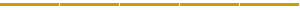FIB_CALC - The Fibonacci calculator. This software package is to be used for real time or historical Fibonacci retrace and expansion objectives. The software prompts the user for nodes, which must consist of two or three per string. Calculated from this information will be the profit expansions: a contracted objective price (COP) utilizing the Fibonacci ratio 0.618, the normal objective price (NOP) utilizing the Fibonacci ratio 1.0, and the expanded objective price (XOP) utilizing the Fibonacci ratio 1.618. Retrace objectives are calculated as well. All calculations with this tool are performed instantly, for fast user-friendly results. Example of typical calculation: Low 1000 to High 2000 to Low 1500 (a 50.00% move) expansions =>   NOP - 2500.0 , COP - 2118.0 , XOP - 3118.0 High 2000 to Low 1500 retraces =>   .382% - 1691.0 , .50% - 1750.0 , .618% - 1809.0 In this sample calculation, at the prompts to enter nodes, 1000, 2000 and 1500 were entered in that sequence. The calculator shows the low 1000 to high 2000 and back to low 1500 and gives the % move based on these three points. The Fibonacci objectives and retraces are calculated based on this inputRevised on 14 October 2001 © 1998 - 2001 Tierra del Fuego Ltd.